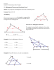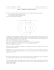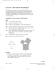# See details. - Math Awareness Month

## Transcription

See details. - Math Awareness Month
```EXPLANATION OF FIGURES for MATH AWARENESS MONTH
Fern Y. Hunt
October 28, 2014
Given an equilateral triangle and any interior point 𝑃, let 𝑑1 , 𝑑2 , 𝑑3 be the
distances (length of the perpendicular) to each side of the triangle (see Figure 1). Then
the sum of the distances 𝑑1 + 𝑑2 + 𝑑3 = ℎ, where ℎ is the length of the altitude of the
triangle. This fact (theorem) was discovered by Vincenzo Viviani, a 17th century Italian
mathematician from Florence. He was also Galileo’s last pupil!
Let’s assume that ℎ = 1. Then we have 𝑑1 + 𝑑2 + 𝑑3 = 1. By allowing
values 𝑑𝑖 to be zero, points on the boundary of the triangle can also be included. Thus
every point in the triangle can be associated with a triple of non-negative numbers that
sum to 1. Although these numbers seem mysterious, in fact they can be calculated if we
know the ordinary (𝑥, 𝑦) coordinates of the point 𝑃.The formulas that one would use can
be derived using some planar geometry. Consequently we can think of functions of 3
variables as functions that are defined on the equilateral triangle, when the variables are
non-negative numbers that sum to 1. This situation arises frequently in many areas of
science and engineering- chemistry, metallurgy, genetics and economics just to name a
few. The translation from mathematics to applications often comes from understanding
how a function changes when variables change. Visualization of the function’s surface is
an important tool for gaining this understanding. This poster shows an example of one
such function that arose in the author’s own work.
Imagine two towns with traffic routes joining them along 3 possible routes
(in the original problem the towns were locations in a network) . Individual cars or trucks
choose route 1 at random with probability 𝑑1 , route 2 with probability 𝑑2 and route 3
with probability 𝑑3 . Since probabilities are non-negative and since the only travel
between the towns occurs on the 3 routes, the probabilities must sum to 1. The function
of interest is the entropy (not the thermodynamic quantity) , 𝐻(𝑑1 , 𝑑2 , 𝑑3 ) =
−(𝑑1 log(𝑑1 ) + 𝑑2 log(𝑑2 ) + 𝑑3 log(𝑑3 )). Figure 2 shows the surface height and level
curves of 𝐻 plotted over the equilateral triangle with altitude 1. If 𝑃 is a point in the
triangle , the height of the surface above the point is 𝐻(𝑑1 , 𝑑2 , 𝑑3 ) the value of 𝐻
1 1 1
corresponding to the coordinates of 𝑃. The point with coordinates �3 , 3 , 3� is at the
1 1 1
center of the triangle (see the pink dot). Its value 𝐻 �3 , 3 , 3� = log(3) is the highest point
on the surface and represents the maximum value of the function. . As 𝐻 decreases, the
surface heights decrease and the size of the corresponding level curves are ovals of
increasing size until a critical value 𝐻 = log(2) is reached (the pink oval), the maximum
value of 𝐻 if there are just 2 roads (i.e. when one of the roads is eliminated). The
probability values where this happens are the coordinates of the points where the log(2)
level curve is tangent to the sides of the triangle. Note that in this case one of the
coordinates is zero. For 𝐻 < log (2), for each level, there are 3 disconnected curves each
one intersecting a side of two triangles. This is reflected in the discontinuity in the curve
in the triangle and the surface itself. More discussion of this can be found at the website.
Finally at 𝐻 = 0, the level curve reduces to 3 points, the vertices of the triangle,
corresponding to the selection of one route and the exclusion of the other two.
Credits for images: Created by Terence Griffin with data supplied by Fern Hunt
Acknowledgement: The author is grateful to Dan Kalman of American University who
REFERENCES:
Posamentier, Alfred S, and Ingmar Lehmann. The Secrets of Triangles: A Mathematical
Journey. Amherst, N.Y: Prometheus Books, 2012. pp 20-21, 77-78.
For a proof without words of Viviani’s theorem see:
Wolf, Samuel, "(No title)", Mathematics Magazine, Vol. 62, no. 3 (1989), p. 190
There are interesting visual enhancements of this proof on the MAA website:
http://www.maa.org/publications/periodicals/convergence/proofs-without-words-andbeyond-proofs-without-words-20
accessed October 29, 2014
Information about the theorem and its applications can be found in the cited Wikipedia
articles.
http://en.wikipedia.org/wiki/Viviani%27s_theorem accessed October 29, 2014, 12:10pm
http://en.wikipedia.org/wiki/Vincenzo_Viviani accessed October 29, 2014 , 12:11pm
P
Figure 1
Figure 2
```

### Pythagoras’ Theorem Now have a go yourself .. . .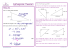### MA.7.A.1.3: Scale Factor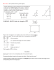### Class 9th Holiday Home Work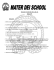### class vii holidays homework - Raja Ram Mohan Roy Public School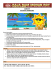### Incenter vs Circumcenter Triangle Worksheet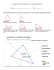### Practice 1.3 KEY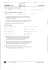### Triangle Play Balls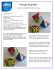### wizBRAIN - W4Kangoeroe### Problem Solving with Pattern Blocks Overview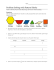### HSM12CC_GM_06_09_CM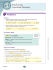### An example on five classical centres of a right angled triangle, pdf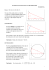### Workshop on âAssessing the Impacts of Public Research Systemsâ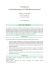### July 11 Newsletter - PCEA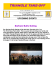### Summative Assessment-I Topper Sample Paper - 8 MATHEMATICS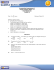### puzzler - Brain Quest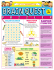### 7.3 Finding Volume Using Cross Sections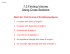### THE AREA OF A TRIANGLE (Do you believe the formula?)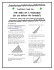### Review for Chapter 8 Test Transformations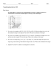### Algebra with shapes How to . . .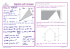### 5.1 Midsegment Theorem and Coordinate Proof